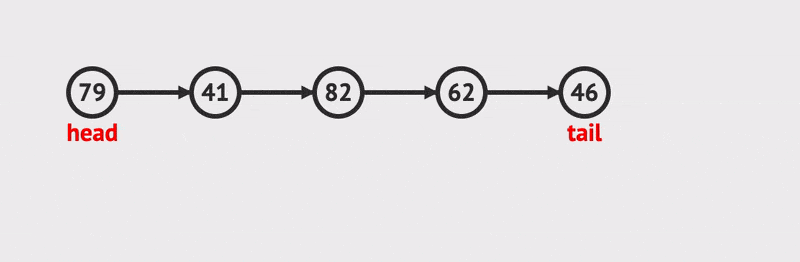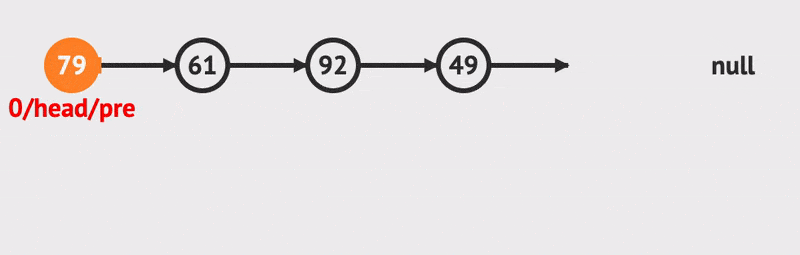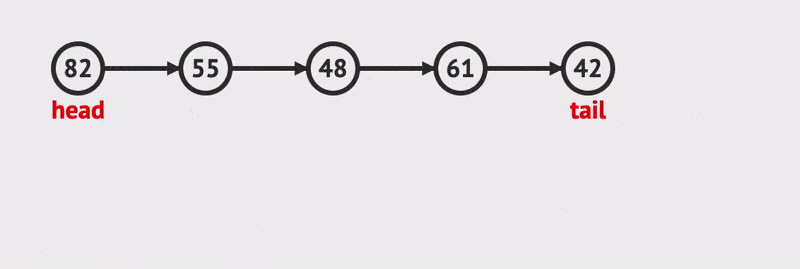Implement a Linked List Coding Interview Question | Skilled.dev
Interview Question

## Course launching November 17, 2020

The most common way to be asked a linked list interview question is to be given the head node of a singly linked list. Since you are only given the head, you know that you don't have access to a wrapper class or the tail node, so all operations must happen by iterating from the beginning.

``````class Node {
constructor(data) {
this.data = data;
this.next = null;
}
}
``````

When you are given this node as an input, it will already be instantiated and have a value for its `data` and `next`.

Instead of creating a special linked list object, we will write functions that take a head node and perform a `prepend`, `append`, `insert`, and `delete` operation that will be how you will use them in an interview. See the final implementation in a REPL.

## Prepend and Append

For `prepend`, we just want to insert a value before the head node we are given, and we can do this in constant O(1) time.Credit: VisuAlgo
``````// O(1) prepend
const prepend = (head, data) => {
// Create a new node to prepend
const prependedNode = new Node(data);
// Set its next to the current node

// Return our new node
return prependedNode;
}

// 42 -> 9000 -> 10 -> 20

// 48 -> 2 -> 9000 -> 10 -> 20
``````

Note that this is one of the advantages of a linked list over an array. When you insert an item, you don't have to shift anything beyond it. All that needs to be done is to update pointers.

For `append`, we want to insert the value at the end. Since we don't have access to the tail, we must iterate through all `n` items until we reach the end. We know we have reached the tail when a node's `next` value is `null`.Credit: VisuAlgo
``````// O(n) append
const append = (head, data) => {
// Create a new node to append
const appendedNode = new Node(data);
// Start at our current node as the head

// Iterate through our list until we reach the last node
while (currentNode.next !== null) {
// Step through nodes
currentNode = currentNode.next;
}

currentNode.next = appendedNode;

// Return our new node
}

// 42 -> 9000 -> 10 -> 20

// 42 -> 9000 -> 10 -> 20 -> 8
``````

Note: If we did have access to the `tail`, we could also append in O(1) time.Credit: VisuAlgo

## Delete a Node

To delete a node, first we find the node that precedes it in the linked list. Then we change this preceding node's `next` to reference the node after the one we want to delete (`precedingNode.next = precedingNode.next.next`). Because no items in the linked list point to the node we wanted to delete any longer, it is effectively removed from our linked list, and the garbage collector will recognize this and clear it from memory.Credit: VisuAlgo
``````// The input is the index number, and the output will be the head node.
const deleteNode = (head, index) => {
// If we want to delete the current head node,
// we just return the node after the current head.
if (index === 0) {
}

// Find the node that precedes the index
// of the node that we want to delete
for (let i = 0; i < index - 1; i++) {
precedingNode = precedingNode.next;
}

// Skip past our node to be removed
// Equivalent to precedingNode.next = precedingNode.next.next
const nodeToBeRemoved = precedingNode.next;
precedingNode.next = nodeToBeRemoved.next;

// There are now no nodes in the linked list that are pointing to nodeToBeRemoved.
// The node that was before it is now pointing to the one after it.

};

// 42 -> 9000 -> 10 -> 20

// 42 -> 9000 -> 20

// 9000 -> 20
``````

## Insert an Item

To insert a node into a linked list, it requires O(n) time because we must iterate through the list to the index that we want to insert at. While this isn't incredibly common to use in interviews, it's good to learn for a holistic understanding of linked lists.Credit: VisuAlgo
``````const insertNode = (head, index, value) => {
// First check if we want to add a node at the head
if (index === 0) {
// Inserting at 0 is equivalent to prepending
}

// Create the node we want to insert
const insertedNode = new Node(value);

// Find the node that precedes the index
// of the node that we want to insert
for (let i = 0; i < index - 1; i++) {
precedingNode = precedingNode.next;
}

// Store the node that should come after the one we insert
const nodeAfterInserted = precedingNode.next;

// Insert our new node at the desired index
precedingNode.next = insertedNode;

// Set the inserted node's `next` pointer
insertedNode.next = nodeAfterInserted;

};

// 42 -> 9000 -> 10 -> 20

// 42 -> 9000 -> 777 -> 10 -> 20
``````

It is not as likely in an interview to be given a fully implemented linked list wrapper class, but it's good to understand it for clarity or if you are asked to implement it yourself.

The following is the implementation for a doubly linked list that has access to the `tail`. Since it is doubly linked, nodes will have a `prev` pointer. We will also track the length which is incremented each time a node is added.

Since we're using a wrapper object, it manages the `head` and `tail` for us.

You can interact with this [doubly linked list wrapper class in a REPL.

``````class DoublyNode {
constructor(data) {
this.data = data;
this.next = null;
this.prev = null;
}
}

constructor(data) {
const initialNode = new DoublyNode(data);

this.length = 0;
this.tail = initialNode;
}

// O(1)
prepend(data) {
const prependedNode = new DoublyNode(data);

this.length++;

}

// O(1)
append(data) {
const appendedNode = new DoublyNode(data);
const previousTail = this.tail;

this.tail = appendedNode;
this.tail.prev = previousTail;

previousTail.next = this.tail;

this.length++;

return appendedNode;
}
}
``````

Our wrapper now tracks the `head` and `tail` nodes. When we `append`/`prepend`, we need to make sure we update our `head` or `tail` and also set the `next` and `prev` correctly. These can all be done in constant time O(1).

## Recursive Iteration

We have used a `while` loop to iterate through our linked lists. Anytime we see a `while` loop, this is a clue that recursion would also work well. Let's see how to iterate a linked list recursively:

``````const findTailNodeRecursively = node => {
// Stopping condition when we find the tail node.
// We know that the `next` will be null for the tail.
if (node.next === null) {
return node;
}

// Continue call our function recursively with the `next` node.
return findTailNodeRecursively(node.next);
};